Technology

# Dynamic Merge in Snowflake using Stored Procedure and Python – For SCD Type 1Creating Snowflake Account

We can create a 30-day free trial account using below link.

https://signup.snowflake.com/

SCD Type 1

In short, keeps the latest the latest data only and old data is overwritten.

SCD Type 1 in Snowflake

Let us consider we have a scenario to load the data into Target table from the Source table.

We can use a normal MERGE statement like below to either UPDATE a record (if exist already based on the key column) else INSERT that record.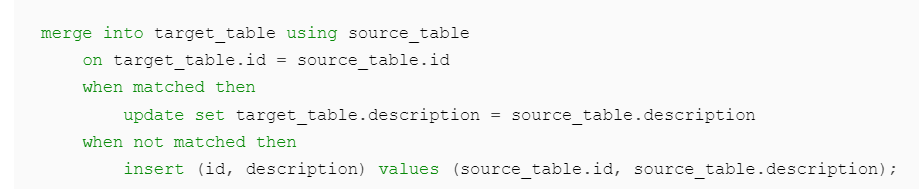Dynamic Merge in Snowflake

When we have to deal with multiple MERGE statement, instead of writing MERGE several times we can leverage Stored Procedure. Along with that let us use an intermediate table (we can call it as ‘Map Table’) that contains the field level mapping across source/target tables.

How to perform Dynamic Merge? (SCD 1)

Define your source – EMP_STAGE and target – EMPLOYEE tables as below.

EMP_STAGE Table look like below.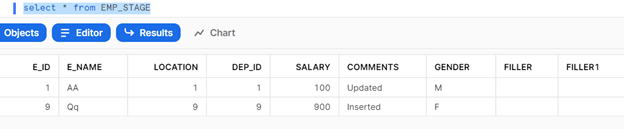Employee Table look like below.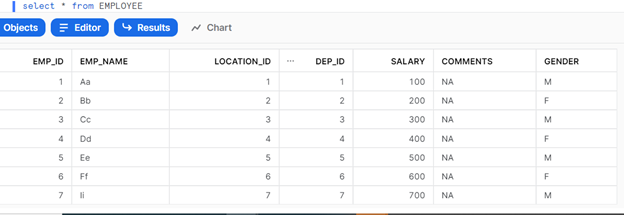Below is how the Employee table will look like once MERGE is completed.

EMP_ID 1 is updated while EMP_ID 9 is inserted here from EMP_STAGE.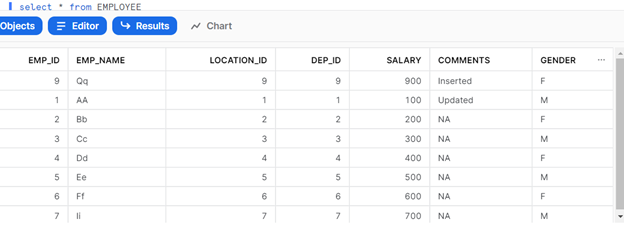MAP Table

Define your field level mapping between Source and Target in Map Table as below.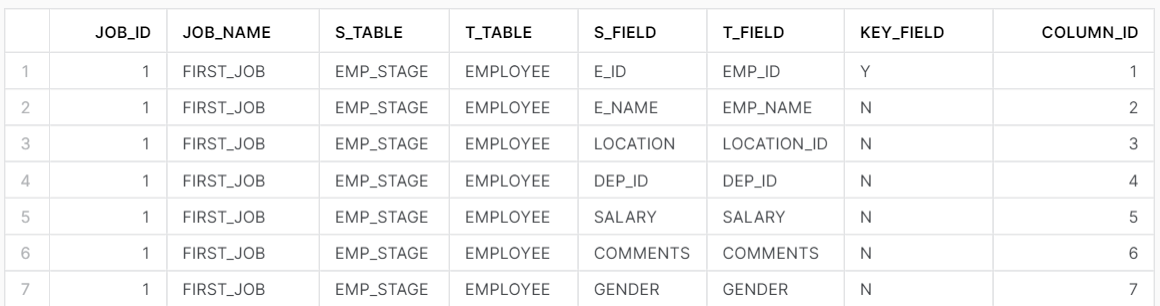KEY_FIELD – Y denotes the key field which can be used to join/compare between 2 tables while KEY_FIELD – N means it’s a non-key field.

Our Scenario: Source table is EMP_STAGE while Target table is EMPLOYEE with Column ID 1 – Employee ID as the only key field. So, have marked ‘Y’ in KEY_FIELD.

Creating Stored Procedure

I have used Python as scripting language and made use of ‘Pandas’ dataframe. We have to declare the version, packages and function name ‘run’ in Handler. The Handler marks as the entry point for our program which is defined after that using ‘def run’.

I have just used 2 parameters here for the stored procedure– one for source and one for target table name.

```CREATE OR REPLACE PROCEDURE AUTO_MERGE(S_TABLE STRING, T_TABLE STRING)
RETURNS STRING
LANGUAGE PYTHON
RUNTIME_VERSION = '3.8'
PACKAGES = ('snowflake-snowpark-python','pandas')
HANDLER = 'run'
AS
\$\$
from snowflake.snowpark.functions import col, lit
from snowflake.snowpark.types import StringType
import pandas as pd

def run(session, s_tab, t_tab):```

NOTE: To use Pandas, you have to enable it first by following the steps mentioned in snowflake documentation else you get an error message to enable Anaconda Package. Please let me know if you still need assistance here.

Stored Procedure Logic

Step 1 is to get the ‘ON’ condition in MERGE statement which is by querying the KEY_FIELD – Y in the MAP Table based on the parameter source/target table names. Then store the result set in Snowflake Dataframe – df (which will look like a normal table).

```def run(session, s_tab, t_tab):
df = session.sql(f'''
SELECT '{s_tab}'||'.'||S_FIELD||'='||'{t_tab}'||'.'||T_FIELD as FILTERS FROM TEST.PYTHON.MAP_TABLE WHERE S_TABLE='{s_tab}' AND T_TABLE='{t_tab}' AND KEY_FIELD='Y'
''')
cc = df.count();
var_filter = '';
df1 = df.to_pandas()
df1['colB'] = " AND ";
df1.iloc[cc-1, 1] = " "
df1['FILTER'] = df1['FILTERS']+df1['colB']
df2 = df1['FILTER']
arr = df2.to_numpy()
arr_key_fields = ''.join(arr);```

Secondly am getting the count of the dataframe and converting it to Pandas Dataframe (df1) to leverage the benefits of Pandas.

I have created a new column ‘colB’ in the dataframe to store the value ‘ AND’. The next line-iloc is used to add blank ‘ ‘ in colB for last record. Then I concatenated on the field names with ‘ AND ’ (last record will not have ‘AND’) and convert this into a Series and then to a numpy array followed by joining it with a blank. This way you can create all the strings which was present in multiple rows into one string to build the ON condition for the MERGE statment.

Note: ‘ AND ‘ is used as a separator for multiple conditions except the last one

 Hey How are You?

Example: Consider above 4 rows & it can be converted into a single string as “Hey How are You?” using the above set of code.

Step 2 is to build the update fields needed for MERGE. Almost the same process but this time I have to filter query based on KEY_FIELD ‘N’ to update the non key fields in MERGE.

```df_update_fields = session.sql(f'''
SELECT '{t_tab}'||'.'||T_FIELD||'='||'{s_tab}'||'.'||S_FIELD as FILTERS FROM TEST.PYTHON.MAP_TABLE E WHERE S_TABLE='{s_tab}' AND T_TABLE='{t_tab}' AND KEY_FIELD='N'
''')
c = df_update_fields.count();
var_filter = '';
df1_update_fields = df_update_fields.to_pandas()
df1_update_fields['colB'] = ", ";
df1_update_fields.iloc[c-1, 1] = " "
df1_update_fields['FILTER'] = df1_update_fields['FILTERS']+df1_update_fields['colB']
df2_update_fields = df1_update_fields['FILTER']
arr_update_fields = df2_update_fields.to_numpy()
arr_update_fields1 = ''.join(arr_update_fields);```

Another difference is I have used comma as separator between various fields. So, I have used the column here colB with comma rather than AND comparing the previous snapshot for ON condition.

Step 3 is to build the insert fields needed for MERGE. It is almost the same process as above but here I am trying to get 2 columns from the MAP_TABLE with no filters.

```df_temp = session.sql(f'''
SELECT T_FIELD, '{s_tab}'||'.'||S_FIELD AS SOURCE FROM TEST.PYTHON.MAP_TABLE WHERE S_TABLE='{s_tab}' AND T_TABLE='{t_tab}' ORDER BY COLUMN_ID
''')
total=df_temp.count()
df_insert=df_temp.to_pandas()

df_insert['colB'] = ", ";
df_insert.iloc[total-1, 2] = " "
df_insert['SRC'] = df_insert['SOURCE']+df_insert['colB']
df_insert['TGT'] = df_insert['T_FIELD']+df_insert['colB']
df_tgt = df_insert['TGT']
df_src = df_insert['SRC']
arr_tgt_col = df_tgt.to_numpy()
arr_tgt = ''.join(arr_tgt_col);
arr_src_col = df_src.to_numpy()
arr_src = ''.join(arr_src_col);```

Step 4 is to build the ON, update and insert strings that we have built so far in a proper MERGE statement string as below in the string mrg_sql. Then run that by displaying the merge sql at last with a success message

```    mrg_sql = "MERGE INTO "
mrg_sql = mrg_sql + f"{t_tab}" + " USING " + f"{s_tab}"
mrg_sql = mrg_sql + " ON " + f"{arr_key_fields}"
mrg_sql = mrg_sql + "WHEN MATCHED THEN UPDATE SET "
mrg_sql = mrg_sql + f"{arr_update_fields1}"
mrg_sql = mrg_sql + "WHEN NOT MATCHED THEN INSERT ("
mrg_sql = mrg_sql + f"{arr_tgt}" + ") VALUES ("
mrg_sql = mrg_sql + f"{arr_src}" + ")"

df = session.sql(f'''
{mrg_sql}
''')
df.collect()
return f"{mrg_sql} is the MERGE QUERY and ran successfully"
\$\$;```

Step 5 is to call the stored procedure with source and target tables as parameters.

CALL AUTO_MERGE(‘EMP_STAGE’,’EMPLOYEE’);

Output

`MERGE INTO EMPLOYEE USING EMP_STAGE ON EMP_STAGE.E_ID=EMPLOYEE.EMP_ID WHEN MATCHED THEN UPDATE SET EMPLOYEE.EMP_NAME=EMP_STAGE.E_NAME, EMPLOYEE.LOCATION_ID=EMP_STAGE.LOCATION, EMPLOYEE.DEP_ID=EMP_STAGE.DEP_ID, EMPLOYEE.SALARY=EMP_STAGE.SALARY, EMPLOYEE.COMMENTS=EMP_STAGE.COMMENTS, EMPLOYEE.GENDER=EMP_STAGE.GENDER WHEN NOT MATCHED THEN INSERT (EMP_ID, EMP_NAME, LOCATION_ID, DEP_ID, SALARY, COMMENTS, GENDER ) VALUES (EMP_STAGE.E_ID, EMP_STAGE.E_NAME, EMP_STAGE.LOCATION, EMP_STAGE.DEP_ID, EMP_STAGE.SALARY, EMP_STAGE.COMMENTS, EMP_STAGE.GENDER ) is the MERGE QUERY and ran successfully`

Before Run – Table SnapshotAfter Run – Table Snapshot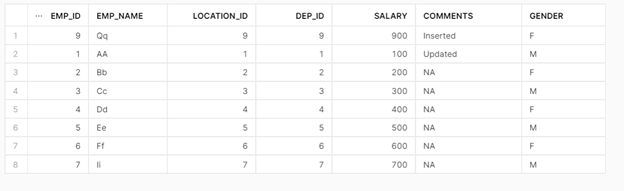Here 1 record (EMP_ID: 9) is inserted and another record (EMP_ID: 1) is updated.

I hope you gained an overview of how we can mix Stored Procedure with Python and Dataframe in Snowflake to auto merge the tables that we need at our ease.

Please share your thoughts and suggestions in the space below and I will do my best to respond to all of them as time allows.

Happy Learning!!

#### Tags

This site uses Akismet to reduce spam. Learn how your comment data is processed.

##### Vignesh Vangapalayam Chidambaram

Vignesh works at Perficient as Technical Architect. He has experience in technologies like Informatica PowerCenter, Databricks, Spark, AWS, and Snowflake. He is keen to learn new technologies.

More from this Author